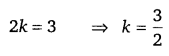# CLASS 9 MATH NCERT SOLUTION FOR CHAPTER – 2 POLYNOMIALS EX – 2.4

## Polynomials

Question 1.
Determine which of the following polynomials has (x +1) a factor:
(i) x+x2 + 1
(ii) x4 + x3+x2+x+1
(iii) x4 + 3x3 + 3x2 + x + 1
(iv) x3 -x2 -(2$\sqrt { 2 }$ )x +$\sqrt { 2 }$

Solution:
The zero of x +1 is -1.

(i) Let p(x) = x3 + x2 + x +1
Then,   p(-1) =  (-1)3 + (-1)2 + (-1) +1
= -1+1-1+1 => p(-1)=   0
So, by the Factor theorem (x +1) is a factor of x3 + x2 + x+1.

(ii) Let p(x)= x4 + x3 + x2 + x +1
Then,   p(-1) = (-1)4 + (-1)3 + (-1)2 + (-1) +1
=1-1+1-1+1 =>      p(-1) =  1
So, by the.Factor theorem (x +1) is not a factor of x4 + x3 + x2 + x +1

(iii) Let p(x) = x4 + 3x3 + 3x2 + x +1
Then,  p(-l) = (-1)4 + 3(-1)3 + 3(-1)2 + (-1) +1
=1-3+3-1+1
=>  P(-1) =  1
So, by the Factor theorem (x +1) is not a factor of x4 + 3x3 + 3x2 + x +1

(iv) Let p(x) = x3-x2-(2 +$\sqrt { 2 }$ )x +$\sqrt { 2 }$
Then, p(-1) = (-1)3 – (-1)2 – (2 +$\sqrt { 2 }$ )(-1) +$\sqrt { 2 }$
= —1—1+2 +$\sqrt { 2 }$ +$\sqrt { 2 }$
= 2$\sqrt { 2 }$
So, by the Factor theorem (x + 1) is not a factor ofx3 -x2 -(2 +$\sqrt { 2 }$ x +$\sqrt { 2 }$

Question 2.
Use the Factor Theorem to determine whether g(x) is a factor of p(x) in each of the following cases :
(i) p(x) – 2x3 + x2 -2x-1, g(x) = x + 1
(ii) p(x) = x3 + 3x2 + 3x + 1, g(x) = x + 2
(iii) p(x) = x3– 4x2 + x + 6, g(x) = x – 3

Solution:
(i) The zero of g(x) = x +1 is x = -1.
Then,  p(-1)= 2(-1)3 +(-1)2 -2(-1)-1
[∵  p(x) = 2x3 + x2 -2x-1]
= -2 +1+2-1 ⇒ p(-1) = 0
Hence, g(x) is a factor of p(x).

(ii) The zero of g(x) = x + 2 is -2.
Then, p(-2) = (-2)3 + 3(-2)2 + 3(-2) +1
[∵ p(x) = x3 + 3x2 + 3x +1]
= -8+12-6 +1 =>p(-2)=-1
Hence, g(x) is not a factor of p(x).

(iii) The zero of g(x) = x – 3 is 3.
Then,p(3) = 33 – 4(3)2 +3 + 6
[∵ p(x) = x3 – 4x2 + x + 6]
= 27-36 +3 + 6 =»       p(3) = 0
Hence, g(x) is a factor of p(x).

Question 3.
Find the value of k, if x -1 is a factor of p(x) in each of the following cases:
(i) p(x) = x2+x + k .
(ii) p(x) = 2x2 + kx + V2=
(iii) p(x) = kx2 -42x + 1
(iv) p(x) = kx2 -3x + k

Solution:Question 4.
Factorise:
(i) 12x2 – 7x+1
(ii) 2x2+7x+3
(iii) 6x2+5x-6
(iv) 3x2-x-4

Solution:

Question 5.
Factorise:
(i) x3-2x2-x+2
(ii) x3-3x2-9x-5
(iii) x3-13x2+32x+20
(iv) 2y3+y2-2y-1

Solution:
(i) Let p(x) = x3-2x2-x+2, constant term of P(x) is 2.
Factors of 2 are ± 1 and ± 2.
Now,  p(1)=13-2(1)2-1+2
=1-2-1+2
By trial we find that p(l) = 0, so (x -1) is a factor of p(x).
So, x3-2x2-x+2= x3-x2-x2 + x-2x+2
= x2(x-1)-x(x-1)-2(x-1)
= (x—1)(x2 -x-2)
= (x -1)(x2 -2x + x -2)
= (x -1) [x(x -2) + l(x -2)]
= (x-1)(x-2)(x+1)

(ii) Let  p(x)= x3 -3x2 -9x-5
By trial, we find that p(5) = (5)3 – 3(5)2 – 9(5) – 5
= 125 – 75 – 45 – 5 = 0
So, (x – 5) is a factor of p(x).
So, x3-3x2-9x-5=x3-5x2+2x2 -10x + x – 5
= x2 (x – 5) + 2x(x – 5) + 1(x – 5)
= (x – 5) (x2+2x+1)
= (x – 5) (x2 + x + x +1)
= (x + 5) [x(x +1) + 1(x +1)]
= (x-5)(x +1) (x +1)
= (x – 5)(x +1)2

(iii) Let p(x)= x3 +13x2 +32x +20
By trial, we find that p(-1) = (-1)3 +13(-1)2 + 32(-1) + 20
= -1 +13 -32 + 20 = -33 + 33 = 0
So (x +1) is a factor of p(x).
So, x3 + 13x2 + 32x + 20 = x3 + x2 + 12x2 + 12x + 20x + 20
= x2 (x +1) + 12x(x +1) + 20(x +1) = (x+1)(x2 + 12x + 20)
= (x+1)(x2 + 10x + 2x + 20)
= (x +1)[x(x +10) + 2(x +10)]
= (x +l)(x +10)(x + 2)

(iv) Let p(y) = 2y3 + y2 – 2y -1
By trial we find that p(l) = 2(1)3 + (1)2 -2(1) -1,
=2+1-2-1=0 So (y -1) is a factor of p(y).
So, 2y3 + y2 -2y-1 = 2y3 -2y2 +3y2 -3y + y-1
= 2y2(y-1) + 3y(y-1) + 1(y-1)
= (y – 1)(2y2 +3y + 1)
= (y – 1)(2y2 + 2y + y + 1)
= (y -1) [2y(y +1) + l(y +1)
= (y-1)(y+ l)(2y+ 1)